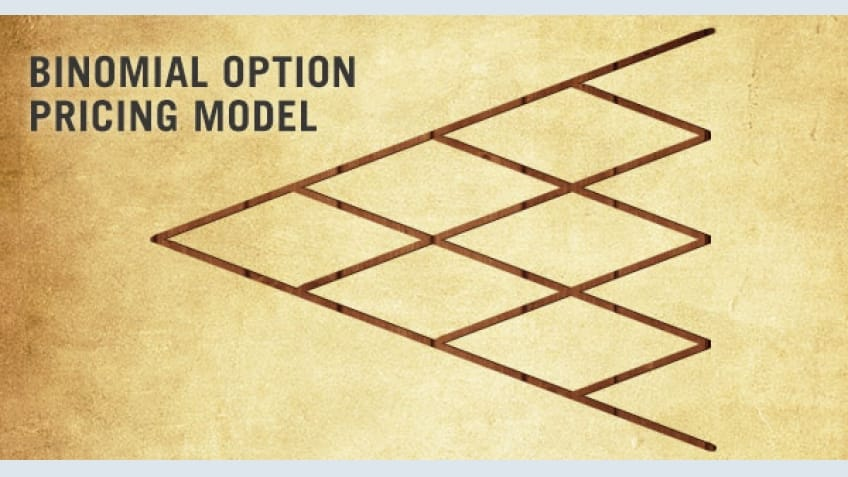Binomial option pricing model is very simple model that is used to price options. When to compared to Black Scholes model and other complex models, binomial option pricing model is mathematically simple and easy to use. This model is based on the concept of no arbitrage. Binomial Option pricing model is an important topic as far as FRM Part 1 exam is concerned. There are both conceptual and numerical questions in exams to test this topic. In this article, I will talk about various concepts related to binomial option pricing model.

### Assumptions in Binomial Option Pricing Model

The assumptions in binomial option pricing models are as follows

1. There are only two possible prices for the underlying asset on the next day. From this assumption, this model has got its name as Binomial option pricing model (Bi means two)
2.  The two possible prices are the up-price and down-price
3. The underlying asset does not pay any dividends
4. The rate of interest (r) is constant throughout the life of the option
5. Markets are frictionless i.e. there are no taxes and no transaction cost
6. Investors are risk neutral i.e. investors are indifferent towards risk

### Binomial option model building process

Let us consider that we have a share of a company whose current value is S0. Now in the next month, the price of this share is going to increase by u% (up state) or it is going to go down by d% (down state). No other outcome of price is possible for this stock in next month. Let p be the probability of up state. Therefore the probability of down state is 1-p.Now let us assume that call option exist for this stock which matures at the end of the month. Let the strike price of the call option be X. Now in case, the option holder decides to exercise the call option at the end of month, what will be the payoffs?

The payoffs are given the below diagramNow, the expected payoff using the probabilities of up state and down state. From the above diagram, the expected value of payoff isOnce the expected value of the payoff is calculated, this expected value of payoff has to be discounted by risk free rate to get the arbitrage free price of call option. Use continuous discounting for discounting the expected value of the payoff. FRM Part 1 uses continuous compounding and discounting for all numerical problems on derivatives.

In some questions, the probability of up state is not given. In such case, probability of up state can be calculated with the formulaWhere;

p = up state probability

r = risk free rate

D = Down state factor

u = Up state factor

Using the above the model building process, similar model can be build for multi period options and also for put options.

### Advantages of Binomial Option Pricing Model

1. Binomial option pricing models are mathematically simple to use.
2. Binomial option pricing model is useful for valuing American options in which the option owner has the right to exercise the option any time up till expiration.
3. Binomial option model is also useful for pricing Bermudan options which can be exercised at various points during the life of the option.

### Limitations of Binomial Option Pricing Model

One major limitation of binomial option pricing model is its slow speed. Computation complexity increases in multi period binomial option pricing model.Vivek Sayal